# AP Calculus BC Practice Test 29

### Test Information9 questions27 minutes

Calculator Allowed

1. The area bounded by the curve x = 3y - y2 and the line x = - y is represented by

2. Find the area bounded by the spiral r = ln θ on the interval π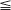θ2π.

3. Write an equation for the line tangent to the curve defined by F(t) = (t2 + 1,2t) at the point where y = 4.

4. Which infinite series converge(s)?

I.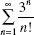II.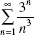III.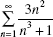5. Bacteria in a culture increase at a rate proportional to the number present. An initialpopulation of 200 triples in 10 hours. If this pattern of increase continues unabated,then the approximate number of bacteria after 1 full day is

6. When the substitution x = 2t - 1 is used, the definite integral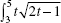dt may be expressed in the form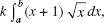where {k. a, b } =

7. The curve defined by x3 + xy - y2 = 10 has a vertical tangent line when x =

8. Use the graph of f shown on [0,7]. Let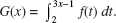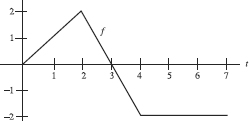G (1) is

9. Use the graph of f shown on [0,7]. LetG has a local maximum at x =